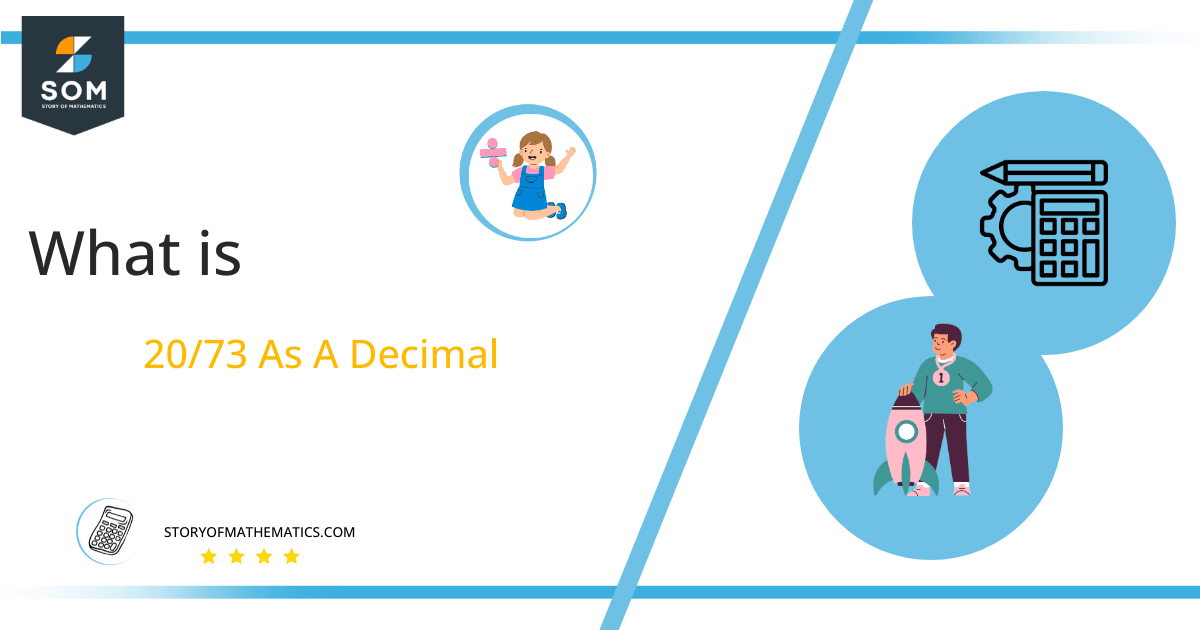# What Is 20/73 as a Decimal + Solution With Free Steps

The fraction 20/73 as a decimal is equal to 0.273972602.

The fraction is represented in p/q form, where is referred to as a numerator, while is referred to as the denominator. Fractions are used to express a connection between two quantities one named as dividend and the other as the divisor. By using a mathematical operator called division, we can convert fractions to decimal values.Here, we are more interested in the division types that result in a Decimal value, as this can be expressed as a Fraction. We see fractions as a way of showing two numbers having the operation of Division between them that result in a value that lies between two Integers.

Now, we introduce the method used to solve said fraction to decimal conversion, called Long Division, which we will discuss in detail moving forward. So, let’s go through the Solution of fraction 20/73.

## Solution

First, we convert the fraction components, i.e., the numerator and the denominator, and transform them into the division constituents, i.e., the Dividend and the Divisor, respectively.

This can be done as follows:

Dividend = 20

Divisor = 73

Now, we introduce the most important quantity in our division process: the Quotient. The value represents the Solution to our division and can be expressed as having the following relationship with the Division constituents:

Quotient = Dividend $\div$ Divisor = 20 $\div$ 73

This is when we go through the Long Division solution to our problem.Figure 1

## 20/73 Long Division Method

We start solving a problem using the Long Division Method by first taking apart the division’s components and comparing them. As we have 20 and 73, we can see how 20 is Smaller than 73, and to solve this division, we require that 20 be Bigger than 73.

This is done by multiplying the dividend by 10 and checking whether it is bigger than the divisor or not. If so, we calculate the Multiple of the divisor closest to the dividend and subtract it from the Dividend. This produces the Remainder, which we then use as the dividend later.

Now, we begin solving for our dividend 20 which after getting multiplied by 10 becomes 200.

We take this 200 and divide it by 73; this can be done as follows:

200 $\div$ 73 $\approx$ 2

Where:

73 x 2 = 146

This will lead to the generation of a Remainder equal to 200 – 146 = 54. Now this means we have to repeat the process by Converting the 54 into 540 and solving for that:

540 $\div$ 73 $\approx$ 7

Where:

73 x 7 = 511

This, therefore, produces another Remainder which is equal to 540 – 511 = 29. Now we must solve this problem to Third Decimal Place for accuracy, so we repeat the process with dividend 290.

290 $\div$ 73 $\approx$ 3

Where:

73 x 3 = 219

Finally, we have a Quotient generated after combining the three pieces of it as 0.273=z, with a Remainder equal to 71.Images/mathematical drawings are created with GeoGebra.